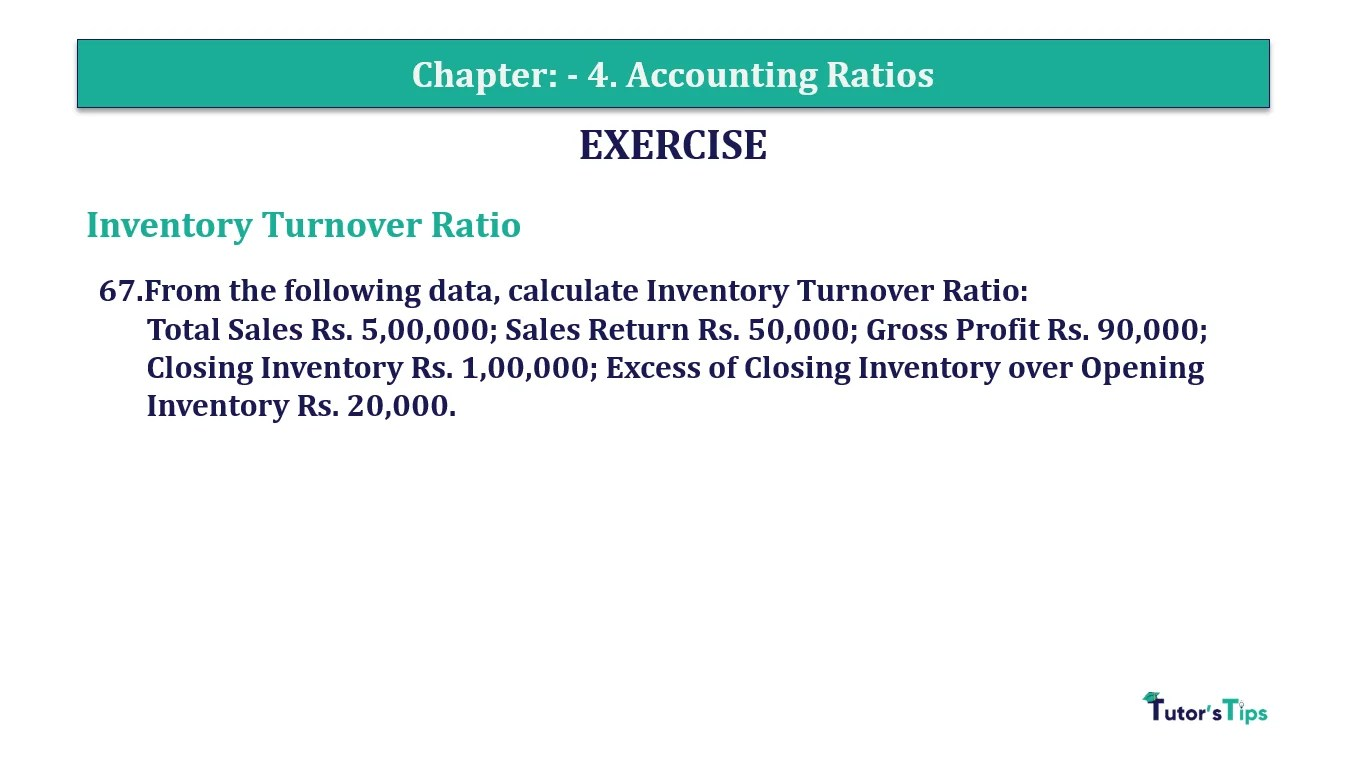# Question 67 Chapter 4 of +2-B – T.S. Grewal 12 ClassQuestion No. 67- Chapter No.4 - T.S. Grewal +2 Book Part B

Question 67 Chapter 4 of +2-B

Interest Coverage Ratio

67. From the following data, calculate Inventory Turnover Ratio:
Total Sales Rs. 5,00,000; Sales Return Rs. 50,000; Gross Profit Rs. 90,000; Closing Inventory Rs. 1,00,000; Excess of Closing Inventory over Opening Inventory Rs. 20,000.

### The solution of Question 67 Chapter 4 of +2-B: –

 Closing Inventory = Rs. 1,00,000 Closing Inventory = Rs. 20,000 + Opening Inventory Opening Inventory = Rs. 1,00,000 – Rs. 20,000 = Rs. 80,000 Cost of Goods Sold = Sales – Sales Return – Gross Profit = Rs. 5,00,00 – Rs. 50,000 – Rs. 90,000 = Rs. 3,60,000

 Average Inventory = Opening Inventory + Closing Inventory 2

 Average Inventory = Rs. 80,000 + 1,00,000 2 = Rs. 90,000

 Inventory Turnover Ratio = Cost of Goods of Goods Sold Average Stock

 Inventory Turnover Ratio = Rs. 3,60,000 Rs. 90,000 = 4 Times

Balance Sheet: Meaning, Format & Examples

Comment if you have any question.

Also, Check out the solved question of previous Chapters: –